You are going to need to quickly recall the three Pythagorean Identities.  The first one is easy to remember because it's just the Pythagorean Theorem.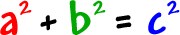on the unit circle.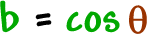But, can you remember the other two?  If you forget, here's the quick way to get them from the first one: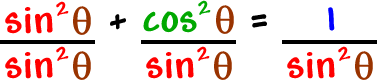(You can also remember that the "co" guys go together!)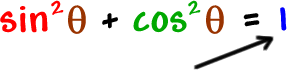Let this one guide you...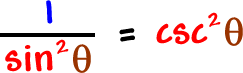So, if you want the guy with a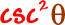, divide by.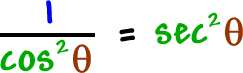So, if you want the guy with a, divide by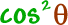.

You'll still be doing a lot of simplifying of trig expressions in Calculus, and these come up a lot!

of 1